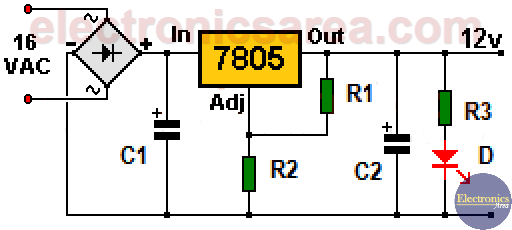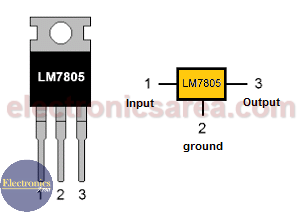# 12 volt Power Supply using 7805

This 12 volt power supply using the 7805 IC is simple and useful. Sometimes we need to get 12 VDC, but we only have 5 or 9 volts regulators (7805, 7809).

This voltage regulator looks like the fixed voltage regulator we already know but with the difference that it have two additional resistors (R1 and R2) (see the image).

## How the 12 volt Power Supply using a 7805 works?

These two resistors are used to establish a voltage divider, which puts a non-zero voltage on the COMMON (ADJ) pin of the 7805 regulator.

Calculating the voltage on the COMMON pin (now ADJ) of 7805, we get 7.2 volts. There are 7.2 volts across the 180 ohm resistor (R2) and 4.8 volts across the 120 ohm resistor (R1).

From the above it follows that the 180 ohm resistor serves to increase the voltage at the COMMON pin. If we want to get a different voltage, we just have to change the value of resistors.Notes:

• We changed the name of the 7805 COMMON pin by the name ADJ because, the voltage on this pin can be changed using external resistors.
• The voltage between the Output pin and the ADJ pin of the regulator, must be maintained at 5 volts (4.8 volts in our case).
• The LM7805 integrated circuit must use a heatsink to prevent any damage.LM7805 voltage regulator Pinout

## List of components for the 12 volt power supply

• 1  diode bridge (DB).
• 1  7805 IC voltage regulator.
• 1  120 ohms resistor, 1/4 watt  (R1).
• 1  180 ohms resistor, 1/4 watt (R2).
• 1  220 ohms resistor, 1/4 watt (R3).
• 1  2200uF, (microfarads) 50 volts  electrolytic capacitor (C1).
• 1  10uF, 25 volts electrolytic capacitor (C2).
• 1  red LED (D).
• 1  220/110VAC to 16VAC (not shown on the diagram) transformer to be connected to the diode bridge.
• 1  heatsink for the LM7805 IC.
• 5
•
• 22
•
•
•
•
•
27
Shares
•
27
Shares
• 5
•
• 22
•
•
•
•
Tagged with: